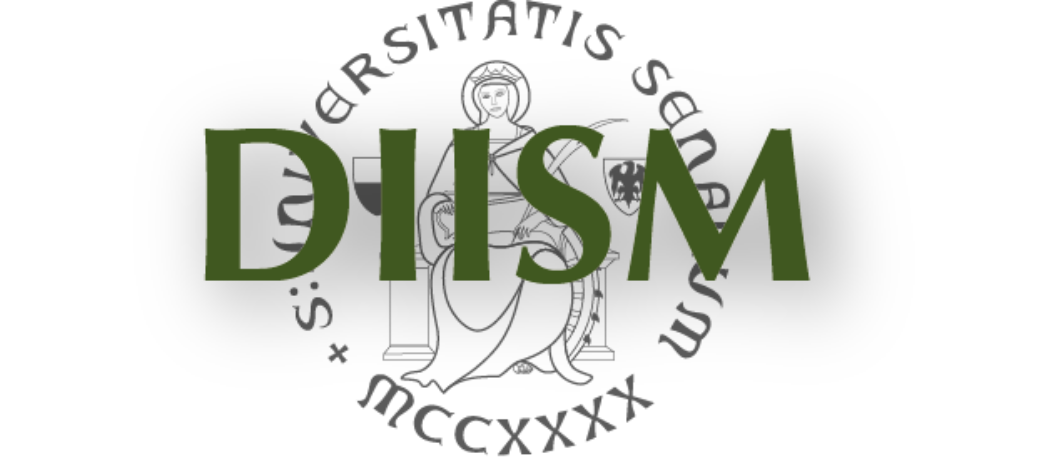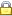DIPARTIMENTO DI INGEGNERIA DELL'INFORMAZIONEE SCIENZE MATEMATICHE UNIVERSITA' DEGLI STUDI DI SIENAPrivacy e Cookie policy

 Research Areas

 Mathematical Logic and Foundations
The area of Mathematical Logic and Foundations of Mathematics includes several research topics, and in particular:

(1)   Theory of Computability.
(2)   Non-Classical Logics, in particular, Many-Valued Logic, Linear Logic and Provability Logic.
(3)   Applications of Algebra to Logic.
(4)   Harmonic theory in the Foundations of Arithmetic.

More specifically, in computablity theory, emphasis is given to research on models of relative computability, with a restricted and limited access to external databases (enumeration reducibility, computing with positive information, etc.); and to the theory of numberings, with a special interest in computations in the Ershov hierarchy, and in the arithmetical hierarchy.
The research on non-classical logic is devoted to some logics which are relevant in Computer Science. For instance, many-valued logic is very useful in the treatment of uncertain information (Ulam game with lies, with applications to error correcting codes, fuzzy control) and in de Finetti's approach to probability in terms of bets.
Linear logic is one of the most prominent logics in the last twenty years, and has deep applications in the theory of computations for providing: (1) geometrical abstraction in both proofs and algorithms, (2) resource-managed calculus machines with polynomial and elementary upper bounds, (3) extensions of the Curry-Howard correspondence in the theory of calculations.
Modal logic is an extension of classical logic by a modality which may have various interpretations, among them, necessity or provability.
The interplay between algebra and logic is a long-standing research topic, which started in Siena in the seventies under the leadership of Roberto Magari. The research then expanded to  algebras for non-classical logics, abstract algebraic logic, universal algebra and categorical algebra.
Finally, the connection, at the origins of mathematics, between number theory and harmonic science has deeply influenced the formation of fundamental arithmetic concepts. These relate in particular to the theory of proportions and means, in connection with the problem of consonance, and the definition of logical and numerical algorithms for the formation of musical scales.

People
Full Professors: Fabio Bellissima, Andrea Sorbi
Assistant Professors: Duccio Pianigiani, Giulia Simi
Research Associates: Francesco Giannini
PhD Students: Cyrille Sandry Simeu

 Harmonic theory in the Foundations of Arithmetic Linear Logic Logic of provability and of interpretability Many-Valued Logic, Algebraic Logic Theory of Computability Theory of Computability, Randomness Universal Algebra, Abstract Algebraic Logic, Categorical Algebra Universal Algebra, Categorical AlgebraUsername: Password:

 Dipartimento di Ingegneria dell'Informazione e Scienze Matematiche - Via Roma, 56 53100 SIENA - Italy